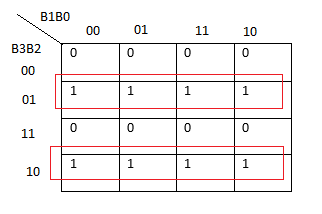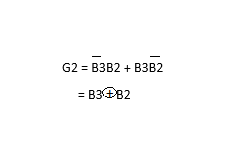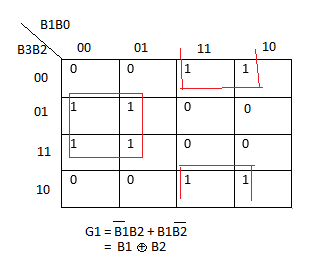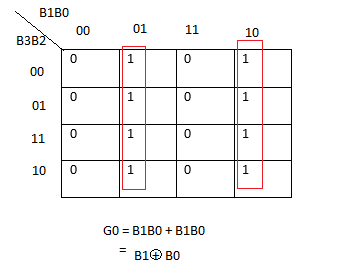# Code Conversion | Binary to Gray Code Converter

In this post on a code conversion, we are going to see Binary to Gray Code conversion using K-map technique.

If you are new to this topic (Digital Logic Circuits), I recommend you to have a look at my previous topic the Study of Logic Gates before reading this topic.

## Binary to gray code converter:

 BINARY INPUT GRAY CODE OUTPUT B3 B2 B1 B0 G3 G2 G1 G0 0 0 0 0 0 0 0 0 0 0 0 1 0 0 0 1 0 0 1 0 0 0 1 1 0 0 1 1 0 0 1 0 0 1 0 0 0 1 1 0 0 1 0 1 0 1 1 1 0 1 1 0 0 1 0 1 0 1 1 1 0 1 0 0 1 0 0 0 1 1 0 0 1 0 0 1 1 1 0 1 1 0 1 0 1 1 1 1 1 0 1 1 1 1 1 0 1 1 0 0 1 0 1 0 1 1 0 1 1 0 1 1 1 1 1 0 1 0 0 1 1 1 1 1 1 0 0 0

A binary code can be converted into a gray code by using this following strategy.

The binary code for 2 is oo10.

The Gray code for 2 is 0011.

Now let us see how to convert binary code to a gray code.

0 +  0 +  1  + 0

0      0      1     1

Firstly, start from the left-hand side and add both bits. so

0+0 = 0

0+0 =0

0+1 = 1

1+0 = 1

If  1+1 = 10 , here 1 is carry and it should be added with next bit.

Now let us do Karnaugh Mapping. (K – map)

Code Conversion | Excess-3 -> BCD converter

K- map for G3:The first preference should be given to 8 pairs so 8 pairs are mapped together in the above k- mapping.

G3 = B3

Code Converter | BCD -> Excess-3 Converter

K- map for G2:Since there is no availability of 8 pairs we cannot map 8 elements. The second preference should be given to 4 so only the 4 elements are mapped together in the k-mapping for G2.K- map for G1:Here also the 4 elements are mapped because there is no possibility for mapping 8 elements. The four elements can be mapped anyway like the one which is shown here. The only thing is it should be adjacent.

Code Converter | Gray Code -> Binary using K-map

K – map for Go:Since there is no availability for 8 elements mapping we can’t map elements. The next preference which is 4 elements mapping that can be done as there is an existence of 2 ( 4 pairs of elements).

Note:

If there is no availability of 4 elements to map, the next preference should be given to 2 elements.

If there is no availability of 2 elements to map, the next preference should be given to 1 element.

logic gates | logic gate | symbols logic diagram | truth table

## Logic Diagram:That’s the process on Binary to Gray Code Converter.

You may also like:

Process | wind power plant

Concept | Thermal Power Plant

Nuclear Power Plant Layout

Concept | Hydro-electric Power Plant

Methods of Neutral Grounding | Neutral Earthing